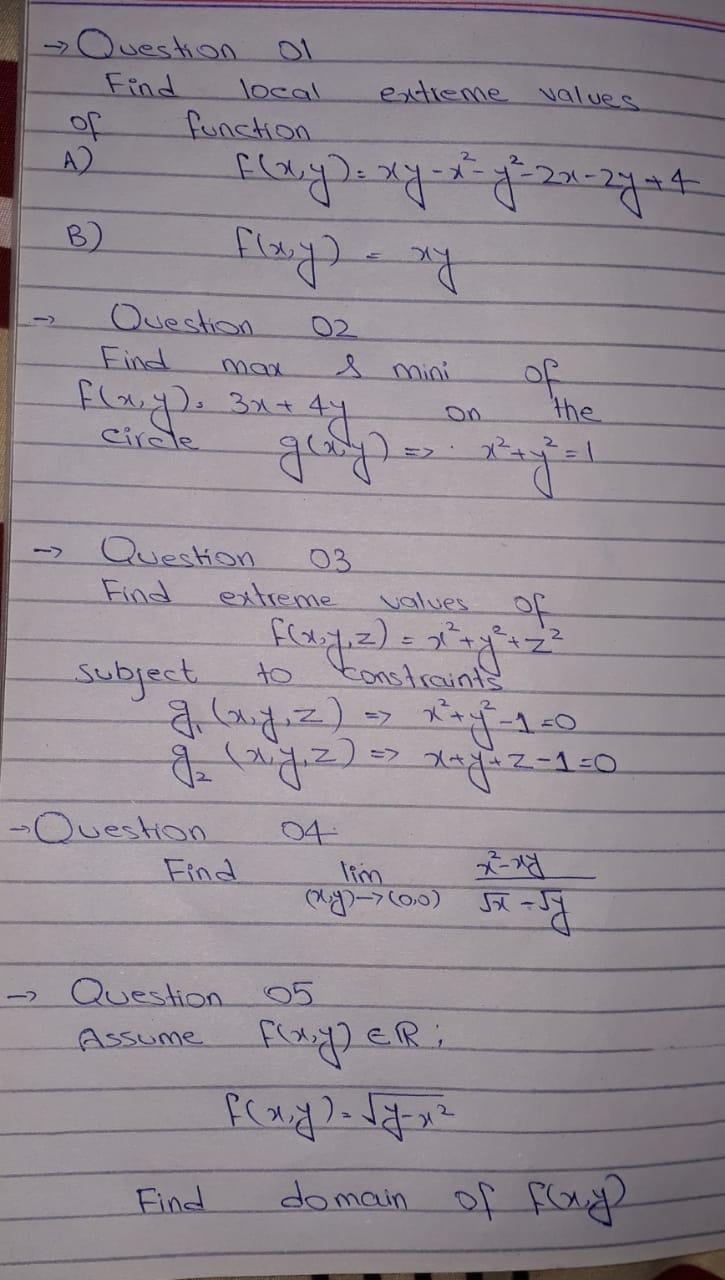### Create an Account

Already have account?

### Forgot Your Password ?

Home / Questions / Question or Find local function A2 extreme values +4 B) Question Find man f(x,y) = xy - x ...

# Question or Find local function A2 extreme values +4 B) Question Find man f(x,y) = xy - x - y = 21-2444 flay) any of the galy) x+y=1 02 s mini 44 fla. y. 3x+ Circle Question 03 Find extreme values of

Question or Find local function A2 extreme values +4 B) Question Find man f(x,y) = xy - x - y = 21-2444 flay) any of the galy) x+y=1 02 s mini 44 fla. y. 3x+ Circle Question 03 Find extreme values of subject to constraints a by,z) a raf-1.0 g (wy, z) = x+y + 2-1=0 Question Find ng (oo) 5x -5 04 lim , Question 05 Assume f(x,y) ER flay) - ly x2 domain of flag Find+++

Apr 15 2021 View more View Less

#### Answer (Solved)Subscribe To Get Solution